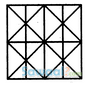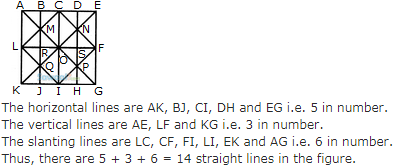Courses

# Non Verbal Reasoning (Odd One Out) MCQ - 3

## 10 Questions MCQ Test General Intelligence and Reasoning for SSC CGL | Non Verbal Reasoning (Odd One Out) MCQ - 3

Description
This mock test of Non Verbal Reasoning (Odd One Out) MCQ - 3 for LR helps you for every LR entrance exam. This contains 10 Multiple Choice Questions for LR Non Verbal Reasoning (Odd One Out) MCQ - 3 (mcq) to study with solutions a complete question bank. The solved questions answers in this Non Verbal Reasoning (Odd One Out) MCQ - 3 quiz give you a good mix of easy questions and tough questions. LR students definitely take this Non Verbal Reasoning (Odd One Out) MCQ - 3 exercise for a better result in the exam. You can find other Non Verbal Reasoning (Odd One Out) MCQ - 3 extra questions, long questions & short questions for LR on EduRev as well by searching above.
QUESTION: 1

### Find the minimum number of straight lines required to make the given figure.Solution:QUESTION: 2

Solution:
QUESTION: 3

### Find the odd one out

Solution:
QUESTION: 4

Find the odd one out

Solution:
QUESTION: 5

Find the odd one out

Solution:
QUESTION: 6

Find the odd one out

Solution:
QUESTION: 7

Find the odd one out

Solution:
QUESTION: 8

Find the odd one out

Solution:
QUESTION: 9

Find the odd one out

Solution:
QUESTION: 10

Find the odd one out

Solution: